# Intermediate Topics in Neural Networks

ModelingNLP/Text AnalyticsNeural Networksposted by Matthew Stewart April 2, 2020

This article is the second in a series of articles aimed at demystifying the theory behind neural networks and how to...This article is the second in a series of articles aimed at demystifying the theory behind neural networks and how to design and implement them for solving practical problems. In this article, I will cover the design and optimization aspects of neural networks in detail.

• Anatomy of a neural network

These tutorials are largely based on the notes and examples from multiple classes taught at Harvard and Stanford in the computer science and data science departments.

I recommend reading the first part of this tutorial first if you are unfamiliar with the basic theoretical concepts underlying the neural network, which can be found here:

# Anatomy of a neural network

Artificial neural networks are one of the main tools used in machine learning. As the “neural” part of their name suggests, they are brain-inspired systems which are intended to replicate the way that we humans learn. Neural networks consist of input and output layers, as well as (in most cases) a hidden layer consisting of units that transform the input into something that the output layer can use. They are excellent tools for finding patterns which are far too complex or numerous for a human programmer to extract and teach the machine to recognize.

While vanilla neural networks (also called “perceptrons”) have been around since the 1940s, it is only in the last several decades where they have become a major part of artificial intelligence. This is due to the arrival of a technique called backpropagation (which we discussed in the previous tutorial), which allows networks to adjust their neuron weights in situations where the outcome doesn’t match what the creator is hoping for — like a network designed to recognize dogs, which misidentifies a cat, for example.

So far, we have discussed the fact that neural networks make use of affine transformations in order to concatenate input features together that converge at a specific node in the network. This concatenated input is then passed through an activation function, which evaluates the signal response and determines whether the neuron should be activated given the current inputs.

We will talk later about the choice of activation function, as this can be an important factor in obtaining a functional network. So far we have only talked about sigmoid as an activation function but there are several other choices, and this is still an active area of research in the machine learning literature.

We also discussed how this idea can be extended to multilayer and multi-feature networks in order to increase the explanatory power of the network by increasing the number of degrees of freedom (weights and biases) of the network, as well as the number of features available which the network can use to make predictions.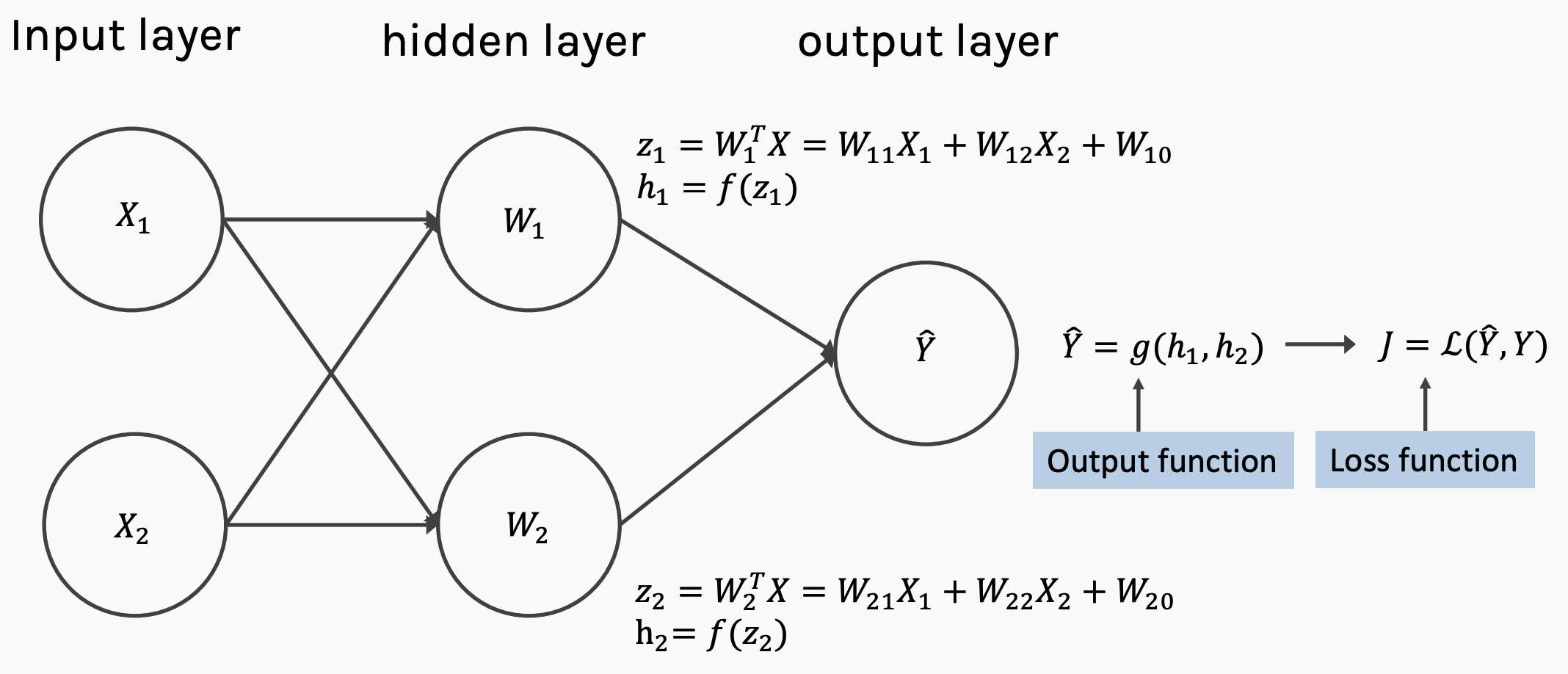A neural network with one hidden layer and two features (the simplest possible multi-layer multi-feature network).

Finally, we discussed that the network parameters (weights and biases) could be updated by assessing the error of the network. This is done using backpropagation through the network in order to obtain the derivatives for each of the parameters with respect to the loss function, and then gradient descent can be used to update these parameters in an informed manner such that the predictive power of the network is likely to improve.

Together, the process of assessing the error and updating the parameters is what is referred to as training the network. This can only be done if the ground truth is known, and thus a training set is needed in order to generate a functional network. The performance of the network can then be assessed by testing it on unseen data, which is often known as a test set.

Neural networks have a large number of degrees of freedom and as such, they need a large amount of data for training to be able to make adequate predictions, especially when the dimensionality of the data is high (as is the case in images, for example — each pixel is counted as a network feature).

A generalized multilayer and multi-featured network looks like this: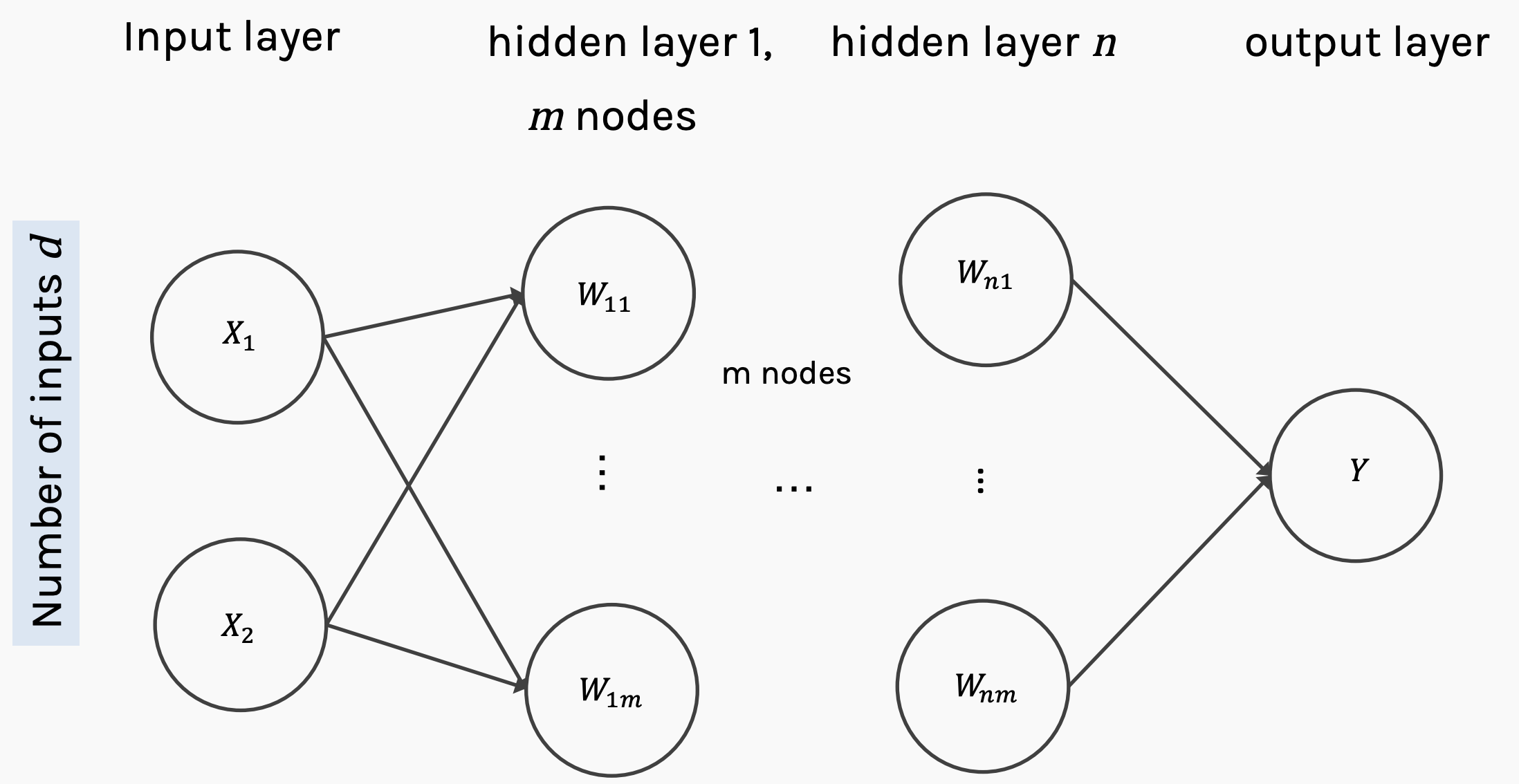Generalized multilayer perceptron with n hidden layers, m nodes, and d input features.

We have m nodes, where m refers to the width of a layer within the network. Notice that this is no relation between the number of features and the width of a network layer.

We also have n hidden layers, which describe the depth of the network. In general, anything that has more than one hidden layer could be described as deep learning. Sometimes, networks can have hundreds of hidden layers, as is common in some of the state-of-the-art convolutional architectures used for image analysis.

The number of inputs, d, is pre-specified by the available data. For an image, this would be the number of pixels in the image after the image is flattened into a one-dimensional array, for a normal Pandas data frame, d would be equal to the number of feature columns.

In general, it is not required that the hidden layers of the network have the same width (number of nodes); the number of nodes may vary across the hidden layers. The output layer may also be of an arbitrary dimension depending on the required output. If you are trying to classify images into one of ten classes, the output layer will consist of ten nodes, one each corresponding to the relevant output class — this is the case for the popular MNIST database of handwritten numbers.

Prior to neural networks, rule-based systems have gradually evolved into more modern machine learning, whereby more and more abstract features can be learned. This means that much more complex selection criteria are now possible.

To understand this idea, imagine that you are trying to classify fruit based on the length and width of the fruit. It may be easy to separate if you have two very dissimilar fruit that you are comparing, such as an apple and a banana. However, this rule system breaks down in some cases due to the oversimplified features that were chosen.

Neural networks provide an abstract representation of the data at each stage of the network which are designed to detect specific features of the network. When considering convolutional neural networks, which are used to study images, when we look at hidden layers closer to the output of a deep network, the hidden layers have highly interpretable representations, such as faces, clothing, etc. However, when we look at the first layers of the network, they are detecting very basic features such as corners, curves, and so on.

These abstract representations quickly become too complex to comprehend, and to this day the workings of neural networks to produce highly complex abstractions are still seen as somewhat magical and is a topic of research in the deep learning community.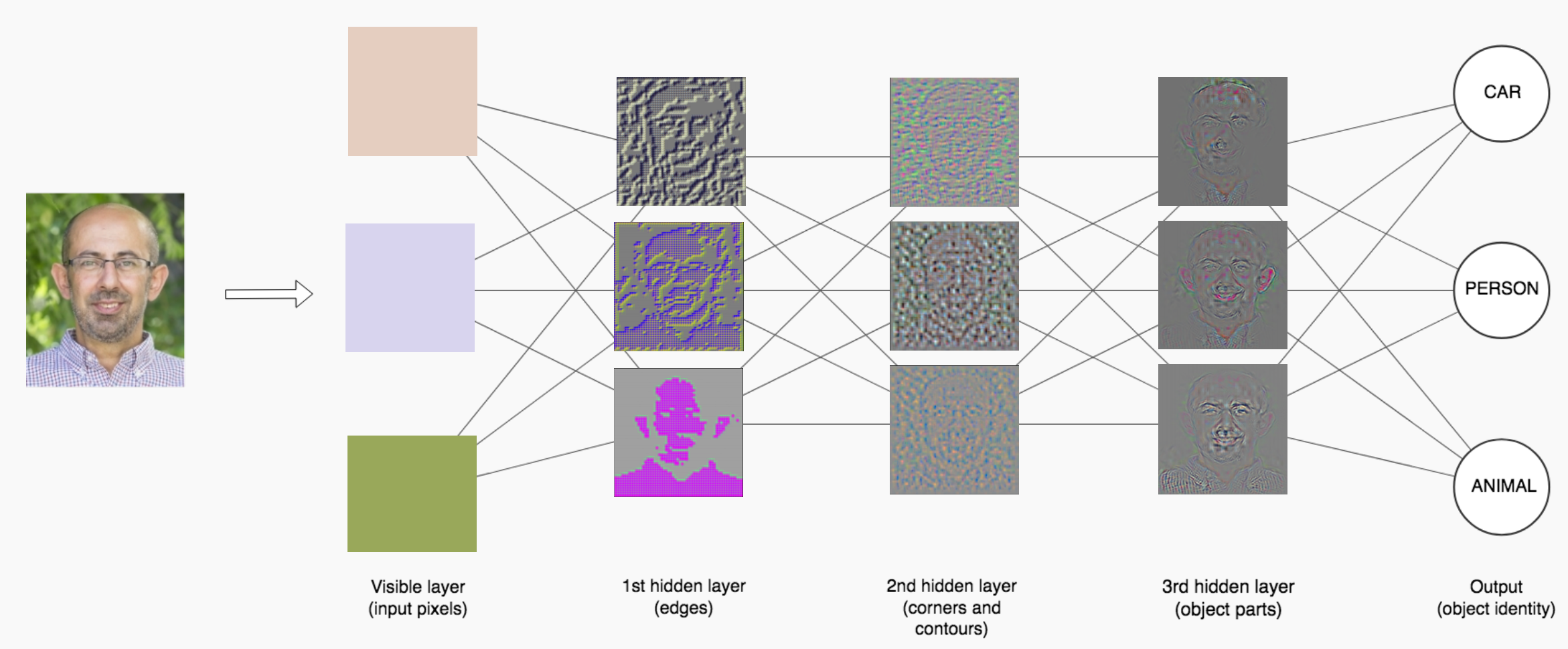An example of a neural network with multiple hidden layers classifying an image of a human face.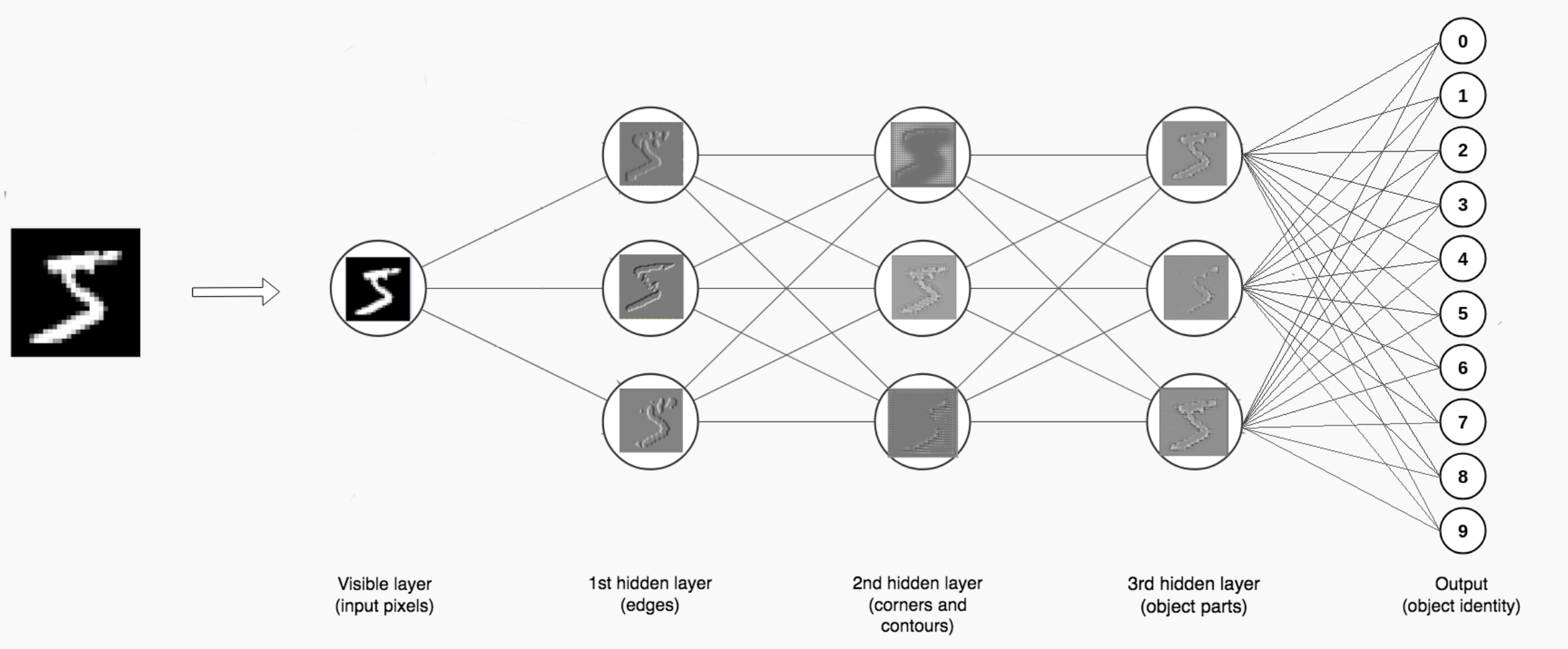An example of a neural network with multiple hidden layers classifying written digits from the MNIST dataset.

We will discuss the selection of hidden layers and widths later. Next, we will discuss activation functions in further detail.

# Activation Functions

Activation functions are a very important part of the neural network. The activation function is analogous to the build-up of electrical potential in biological neurons which then fire once a certain activation potential is reached. This activation potential is mimicked in artificial neural networks using a probability. Depending upon which activation function is chosen, the properties of the network firing can be quite different.

The activation function should do two things:

• Ensures not linearity

The general form of an activation function is shown below: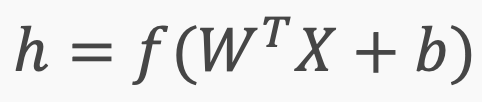f(.) represents the activation function acting on the weights and biases, producing h, the neural output.

Why do we need non-linearity? Technically, we do not need non-linearity, but there are benefits to using non-linear functions.

If we do not apply an activation function, the output signal would simply be a linear functionA linear function is just a polynomial of one degreeNow, a linear equation is easy to solve but they are limited in their complexity and have less power to learn complex functional mappings from data. A neural network without any activation function would simply be a linear regression modelwhich is limited in the set of functions it can approximate. We want our neural network to not just learn and compute a linear function but something more complicated than that.

This goes back to the concept of the universal approximation theorem that we discussed in the last article — neural networks are generalized non-linear function approximators. Using a non-linear activation we are able to generate non-linear mappings from inputs to outputs.

Another important feature of an activation function is that it should be differentiable. This is necessary in order to perform backpropagation in the network, to compute gradients of error (loss) with respect to the weights which are then updated using gradient descent. Using a linear activation function results in an easily differentiable function that can be optimized using convex optimization, but has a limited model capacity.

Why do we want to ensure we have large gradients through the hidden units?

If we have small gradients and several hidden layers, these gradients will be multiplied during backpropagation. Computers have limitations on the precision to which they can work with numbers, and hence if we multiply many very small numbers, the value of the gradient will quickly vanish. This is commonly known as the vanishing gradient problem and is an important challenge when generating deep neural networks.

Some of the most common choices for activation function are:

• Sigmoid

These activation functions are summarized below:

Sigmoid

The sigmoid function was all we focused on in the previous article.

Actually, this function is not a particularly good function to use as an activation function for the following reasons:

• Sigmoids suffer from the vanishing gradient problem.

Sigmoids are still used as output functions for binary classification but are generally not used within hidden layers. A multidimensional version of the sigmoid is known as the softmax function and is used for multiclass classification.

Tanh

The zero centeredness issue of the sigmoid function can be resolved by using the hyperbolic tangent function. Because of this, the hyperbolic tangent function is always preferred to the sigmoid function within hidden layers. However, the hyperbolic tangent still suffers from the other problems plaguing the sigmoid function, such as the vanishing gradient problem.

ReLU and Softplus

The rectified linear unit is one of the simplest possible activation functions. If the input to the function is below zero, the output returns zero, and if the input is positive, the output is equal to the input. ReLU is the simplest non-linear activation function and performs well in most applications, and this is my default activation function when working on a new neural network problem.

As you can see, softplus is a slight variation of ReLU where the transition at zero is somewhat smoothened — this has the benefit of having no discontinuities in the activation function.

ReLU avoids and rectifies the vanishing gradient problem. Almost all deep learning Models use ReLU nowadays. However, ReLU should only be used within hidden layers of a neural network, and not for the output layer — which should be sigmoid for binary classification, softmax for multiclass classification, and linear for a regression problem.

Leaky ReLU and Generalized ReLU

One problem with ReLU is that some gradients can be unstable during training and can die. It can cause a weight update causes the network to never activate on any data point. These are commonly referred to as dead neurons.

To combat the issue of dead neurons, leaky ReLU was introduced which contains a small slope. The purpose of this slope is to keep the updates alive and prevent the production of dead neurons.

The leaky and generalized rectified linear unit are slight variations on the basic ReLU function. The leaky ReLU still has a discontinuity at zero, but the function is no longer flat below zero, it merely has a reduced gradient. The difference between the leaky and generalized ReLU merely depends on the chosen value of α. Thus, leaky ReLU is a subset of generalized ReLU.

Maxout

Maxout is simply the maximum of k linear functions — it directly learns the activation function. It is a hybrid approach which consists of linear combinations of ReLU and leaky ReLU units.

Swish: A Self-Gated Activation Function

Currently, the most successful and widely-used activation function is ReLU. However, swish tends to work better than ReLU on deeper models across a number of challenging datasets. Swish was developed by Google in 2017.

Swish is essentially the sigmoid function multiplied by x:

f(x) = x · sigmoid(x)

One of the main problems with ReLU that gives rise to the vanishing gradient problem is that its derivative is zero for half of the values of the input xThis is problematic as it can result in a large proportion of dead neurons (as high as 40%) in the neural network. Swish, on the other hand, is a smooth non-monotonic function that does not suffer from this problem of zero derivatives.

Swish is still seen as a somewhat magical improvement to neural networks, but the results show that it provides a clear improvement for deep networks. To read more about this, I recommend checking out the original paper on arxiv:

## Searching for Activation Functions

### The choice of activation functions in deep networks has a significant effect on the training dynamics and task…

#### arxiv.org

In the next section, we will discuss loss functions in more detail.

# Loss Functions

Loss functions (also called cost functions) are an important aspect of neural networks. We have already discussed that neural networks are trained using an optimization process that requires a loss function to calculate the model error.

There are many functions that could be used to estimate the error of a set of weights in a neural network. However, we prefer a function where the space of candidate solutions maps onto a smooth (but high-dimensional) landscape that the optimization algorithm can reasonably navigate via iterative updates to the model weights.

Maximum Likelihood provides a framework for choosing a loss function when training neural networks and machine learning models in general. As such, the loss function to use depends on the output data distribution and is closely coupled to the output unit (discussed in the next section).

Cross-entropy and mean squared error are the two main types of loss functions to use when training neural network models.

However, the maximum likelihood approach was adopted for several reasons, but primarily because of the results it produces. More specifically, neural networks for classification that use a sigmoid or softmax activation function in the output layer learn faster and more robustly using a cross-entropy loss function than using mean squared error.

“The use of cross-entropy losses greatly improved the performance of models with sigmoid and softmax outputs, which had previously suffered from saturation and slow learning when using the mean squared error loss.”

— Page 226, Deep Learning, 2016.

Cross-entropy between training data and model distribution (i.e. negative log-likelihood) takes the following form:

Below is an example of a sigmoid output coupled with a mean squared error loss.

Contrast the above with the below example using a sigmoid output and cross-entropy loss.

In the next section, we will tackle output units and discuss the relationship between the loss function and output units more explicitly.

# Output Units

We have already discussed output units in some detail in the section on activation functions, but it is good to make it explicit as this is an important point. It is relatively easy to forget to use the correct output function and spend hours troubleshooting an underperforming network.

For binary classification problems, such as determining whether a hospital patient has cancer (y=1) or does not have cancer (y=0), the sigmoid function is used as the output.

For multiclass classification, such as a dataset where we are trying to filter images into the categories of dogs, cats, and humans. This uses the multidimensional generalization of the sigmoid function, known as the softmax function.

There are also specific loss functions that should be used in each of these scenarios, which are compatible with the output type. For example, using MSE on binary data makes very little sense, and hence for binary data, we use the binary cross entropy loss function. Life gets a little more complicated when moving into more complex deep learning problems such as generative adversarial networks (GANs) or autoencoders, and I suggest looking at my articles on these subjects if you are interested in learning about these types of deep neural architectures.

A summary of the data types, distributions, output layers, and cost functions are given in the table below.

In the final section, we will discuss how architectures can affect the ability of the network to approximate functions and look at some rules of thumb for developing high-performing neural architectures.

# Architecture

In this section, we will look at using a neural network to model the function y=x sin(x) using a neural network, such that we can see how different architectures influence our ability to model the required function. We will assume our neural network is using ReLU activation functions.

A neural network with a single hidden layer gives us only one degree of freedom to play with. So we end up with a pretty poor approximation to the function — notice that this is just a ReLU function.

Adding a second node in the hidden layer gives us another degree of freedom to play with, so now we have two degrees of freedom. Our approximation is now significantly improved compared to before, but it is still relatively poor. Now we will try adding another node and see what happens.

With a third hidden node, we add another degree of freedom and now our approximation is starting to look reminiscent of the required function. What happens if we add more nodes?

Our neural network can approximate the function pretty well now, using just a single hidden layer. What differences do we see if we use multiple hidden layers?

This result looks similar to the situation where we had two nodes in a single hidden layer. However, note that the result is not exactly the same. What occurs if we add more nodes into both our hidden layers?

We see that the number of degrees of freedom has increased again, as we might have expected. However, notice that the number of degrees of freedom is smaller than with the single hidden layer. We will see that this trend continues with larger networks.

Our neural network with 3 hidden layers and 3 nodes in each layer give a pretty good approximation of our function.

Choosing architectures for neural networks is not an easy task. We want to select a network architecture that is large enough to approximate the function of interest, but not too large that it takes an excessive amount of time to train. Another issue with large networks is that they require large amounts of data to train — you cannot train a neural network on a hundred data samples and expect it to get 99% accuracy on an unseen data set.

In general, it is good practice to use multiple hidden layers as well as multiple nodes within the hidden layers, as these seem to result in the best performance.

It has been shown by Ian Goodfellow (the creator of the generative adversarial network) that increasing the number of layers of neural networks tends to improve overall test set accuracy.

The same paper also showed that large, shallow networks tend to overfit more — which is one stimulus for using deep neural networks as opposed to shallow neural networks.

Selecting hidden layers and nodes will be assessed in further detail in upcoming tutorials.

I hope that you now have a deeper knowledge of how neural networks are constructed and now better understand the different activation functions, loss functions, output units, and the influence of neural architecture on network performance.

Future articles will look at code examples involving the optimization of deep neural networks, as well as some more advanced topics such as selecting appropriate optimizers, using dropout to prevent overfitting, random restarts, and network ensembles.

The third article focusing on neural network optimization is now available:

## Neural Network Optimization

Deep learning courses:

NLP-oriented:

Vision-oriented:

Important neural network articles:## Matthew Stewart

Matthew is an environmental and data science Ph.D. student at Harvard University working on developing drone-based sensor systems to study tree emissions in the tropical Amazon rainforest. He is also a part-time machine learning consultant that specializes in computer vision and IoT applications.

1